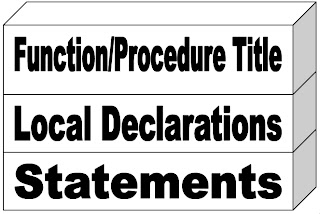Tuesday, 3 August 2010

Function and Procedure

There are two kind of subroutine in Pascal : Function and Procedure. The difference between these subroutines is in return value. Function gives a return value, but procedure doesn’t. The structure of function and procedure are the same :Here is the example of procedure and function :

program FunctionAndProcedure;
{\$APPTYPE CONSOLE}
uses
SysUtils;

procedure Add1(x, y : integer);
var
z : integer;
begin
z := x + y;
writeln(x,' + ',y,' = ',z,' (using procedure)');
end;

function Add2(x, y : integer) : integer;
begin
Add2 := x + y;
end;

begin